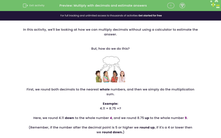# Multiply with decimals and estimate answers

In this worksheet, students perform multiplications by rounding numbers before carrying out the calculation.Key stage:  KS 2

Curriculum topic:   Number: Fractions, Decimals and Percentages

Curriculum subtopic:   Solve Rounding Problems

Difficulty level:#### Worksheet Overview

In this activity, we'll be looking at how we can multiply decimals without using a calculator to estimate the answer.

But, how do we do this?First, we round both decimals to the nearest whole numbers, and then we simply do the multiplication sum.

Example:

4.11 × 8.75 =?

Here, we round 4.11 down to the whole number 4, and we round 8.75 up to the whole number 9.

(Remember, if the number after the decimal point is 5 or higher we round up, if it's a 4 or lower then we round down.)

So, now we have the following sum, which we can work out using our times table knowledge.

4 × 9 = 36

### What is EdPlace?

We're your National Curriculum aligned online education content provider helping each child succeed in English, maths and science from year 1 to GCSE. With an EdPlace account you’ll be able to track and measure progress, helping each child achieve their best. We build confidence and attainment by personalising each child’s learning at a level that suits them.

Get started